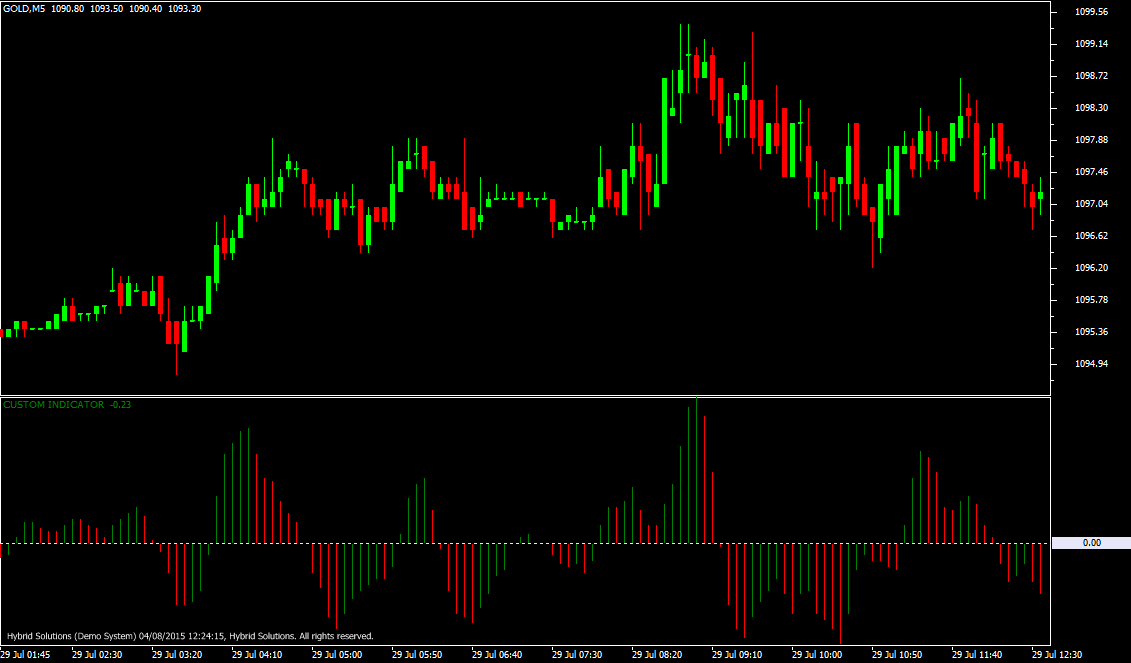# Accelerator

Free

Developed By: NeeduVerdeSolution

In stock

Accelerator is a VTL Indicator useful for determining start and end of trends. It is calculated from the Simple Moving Averages (SMA) of median price of security.

SKU: 606 Categories: ,Accelerator is a client side VTL Indicator useful for determining start and end of trends. It is calculated from the Simple Moving Averages (SMA) of median price of security.

The Accelerator indicator value oscillates around the center (0) line. When the value reaches extreme negative values and is slowly rising, it is a signal to place Long trades (take buy positions) or close Short trades (Close sell positions). Similarly, when the value has reached extreme positive and has started to fall, it is a signal to place short trades (take sell positions) or exit Long trades (Close buy positions).

User Inputs:
a) PERIOD_SHORT – The period of the short term Moving Average.
b) PERIOD_LONG – The period of the long term Moving Average.
c) PERIOD_SMA – The period of the Moving Average of the difference between the short term and long term Moving Average.

At first short term (PERIOD_SHORT) and long term (PERIOD_LONG) Moving Average of the median price are calculated. Then the long term Moving Average is subtracted from the short term Moving Average. Finally, a simple Moving Average (PERIOD_SMA) of this difference is calculated – which is the Accelerator indicator.